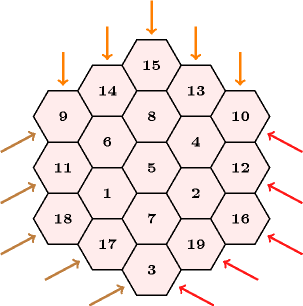### 3.7.140. Magic hexagon

A constraint that can be used for modelling some parts of the magic hexagon problem. The magic hexagon problem, see Figure 3.7.40 for an example, consists of finding an arrangement of $n$ hexagons, where an integer from 1 to $n$ is assigned to each hexagon so that (1) each integer from 1 to $n$ occurs exactly once, (2) the sum of the numbers along any straight line is the same.

##### Figure 3.7.40. A magic hexagon of order 3 filled by integers 1 through 19 where the sum of the integers in each row of cells, in all three directions, is 38